main page   list of papers   library

© Copyright - Karim A. Khaidarov, September 1, 2003. Updated September 16, 2003

# Gravitating Ether

Dedicated to the bright memory of my daughter Anastasia

 That's for thyself to breed another thee, Or ten times happier, be it ten for one; Ten times thyself were happier than thou art, If ten of thine ten times refigured thee: Then what could death do, if thou shouldst depart, Leaving thee living in posterity? Be not self-will'd, for thou art much too fair To be death's conquest and make worms thine heir. William Shakespeare

Taking as fact  ether presence in Universe, united quasiisotropic and elastic medium, being basic matter - the carrier of the whole energy, all objects and processes, occurring in Universe, and taking for basic concepts about it the works of R. Hooke , W. Ritz  and F. Gorgatsevich , we can consider its main characteristics.

Nikitin Phenomenon

Results of precise measurements of coner variations of horizontal light ray which made in conditions of gravimetric point in Sevastopol city on April - May 2003 by George Nikitin are presented on his site http://gnikitin.narod.ru/ (fig. 1).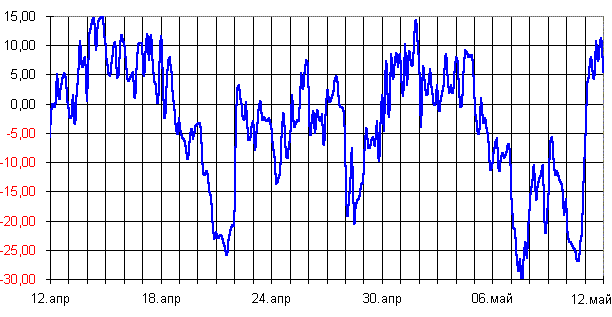Fig.1. Results of measurements of coner variations of horizontal light ray
(vertical scale is seconds of arc) [G. Nikitin, 2003]

Defining by following method that weighted average of ray downwards deflection corresponds to the second space velocity, George Nikitin explains this phenomenon with ray drift by flow of ether, falling to the Earth with this velocity exactly.

Ω = (A + 2K + E) / 4 = - 7.9" ±0.86"

here A - is arithmetic mean of ray drift, equals - 3.1";
K - is mean square of ray drift, equals ±10.43";
E - is algebraic semi-sum of extremal magnitudes, equals -7.6"

The value of drift at Galilean adding of velocities is:

arcsin( VII / c ) = 7.7"

here VII - is second space velocity, equals for the Earth 11.2 [km/s];
c - is light velocity 300,000 [km/s].

Besides that Nikitin discovered intraday fluctuation of horizontal and vertical light ray deflection, having evident relative phase offset (refer to fig.2).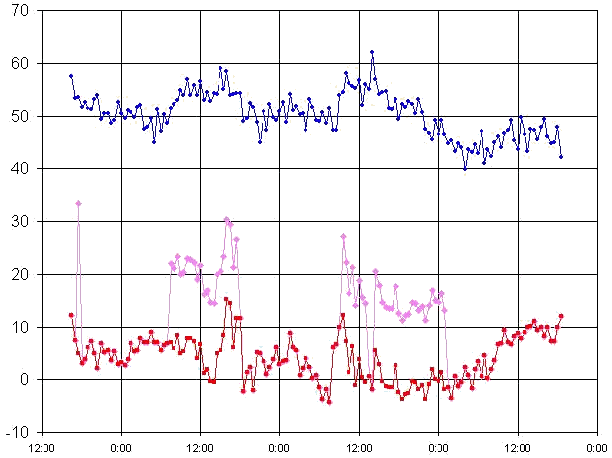Fig.2. Intraday horizontal light ray angle fluctuation (seconds of arc).
Vertical deflections are red, horizontal deflection are blue. [George Nikitin, 2003]

Possible confirm that under ethereal interpreting the drift of ray, data shown on fig.2 have a good fitting with results of classical Michelson's and Miller's experiments. Corners translated in velocity, give ether velocity as several km at second of arc, but phase shift, shows spiral ether captivation by the Earth and explains more high value of velocity for bigger a.s.l. heights.

However, at the ether notion, as some foam, falling from the haven (for instance, look at R.Cahill. - http://www.scieng.flinders.edu.au/cpes/people/cahill_r/ ), it is difficult to interpret other experiments, showing that ether velocity for Earth is 390±30 [km/s]. There are measurements of light velocity made by S. Marinov, known anisotropy of Cosmic Microwave Background, and other astronomical measurements.

Working Ether Model

For tying up inconsistent experimental data, considering that most simplest and the main characteristic of the ether is a gravitation phenomena, we will take following simplified ether model, including particles - carriers of gravity interactions.

We will imagine that the ether consists of dense packed incondensable massless elements - "amers", interactions between which propagate with greatly possible velocity for this medium - velocity of the light.

The gravitation phenomena appears, when the bodies, having a stationary mass, are present in ether. The elementary particles of these bodies continuously and sphere symmetrical "shoot off" dislocations as type of vacancies having velocity of light (ether waves), that are emptinesses. Vacancies are scattering in all sides, exceedingly slowly losing its initial velocity in process of ether fading .

The frequency of forming the ether vacancies strictly proportional to mass, giving birth these vacancies. Naturally, this frequency must be not lesser than Compton frequency. Thereby the following dependency exists for "gravity pulse":

 ωg = 2πmc2/h [s-1], (1)

here m - is mass generated vacancies of the ether,
h - is Planck constant,
c - is light velocity.

So the local decompaction of the ether is formed by vacancies, density of which back proportional to square of distance from source. The detours from this dependency must observed only on cosmological distances, where occurs the slow loss of velocity of these vacancies. We will call these vacancies gravitons.

For the further discourses we will consider the "amer" characteristics.

Having practically endless resistance to compression, but having ability to enlarge with the light velocity aside emptinesses - vacancies, it can base in five main conditions:

• passive, - when it will jam with all sides and no motion in him, except rotations impossible;
• active, - when on a certain distance from it, smaller than radius of interaction, there is vacancy and shearing strain in this volume are possible;
• excitated, - when it is adjacent to vacancy;
• dominant-excited, when the vacancy moving is directed to it;
• displacement, - when amer moves into formed new vacancy.

The velocity of amer or vacancies displacement depends on initial gravity pulse. The vacancies if even disappear, that much seldom. Their time of existance is very great.

On the one hand volume actuated by ether vacancy is defined by light velocity, but on the other hand - by velocity of vacancy, thereby radius, cross-section and volume of interaction of vacancies accordingly proportional to

rv = rg c / v , sv = ( rg c / v )2 , vv = ( rg c / v )3 ,

here rg - is graviton interaction radius v = c, commensurable to vacancy size.
v - is vacancy moving velocity,
c - is light velocity.

Thence we get measly cross-section of graviton interaction, but cross-section of low-velocity vacancies commensurable with square of distance between them. Besides, gravity pulse for low-velocity vacancies much below than graviton pulse:

Iv = Ig (v/c)2

Stated working model allows to do following findings, allowing contradiction between difference of light velocity measurements.

1. Practically whole ether in Universe inheres in active condition since there is possibility of shift waves spreading within ether - waves of the light. That possible explain by presence of pseudo-gas of low-velocity vacancies within ether.
2. Gravitons that are vacancies, radiated by masses at the light velocity, practically do not react with this pseudo-gas and do not influence upon shear and torsion waves (strain) within ether. This is explained the registration flow of only low-velocity vacancies.
3. The ether practically still. Corpuscle velocity of light must have the constancy for ether (390±30 km/with average offset for Earth). We will call it corpusclar ether.
4. The vacancies of ether - ethereal pseudo-gas, led away by masses, is a point of counting out to phase velocity of light. We will call it phase ether.

It is interesting a question about absorption of ether by bodies. Is this only process of transformation of low-velocity vacancies to gravitons, or vacancies are absorbed by bodies regardless of gravitons emission? By other words does the law of "graviton charge" conservation exist?

For that we will consider the process of absorption ethereal pseudo-gas - vacancies by Earth, by reduction of curve, shown on fig.1, to velocity [km/s] and by taking the integral.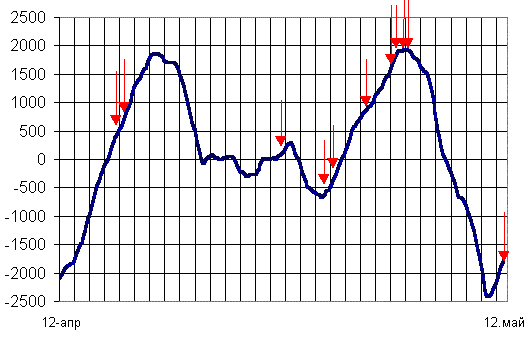Fig.3. Curve of emission-absorption of ethereal pseudo-gas by the Earth at april - may 2003
(Axis of ordinates is volume in trillions km3). The most large earthquakes (M ~ 6)
for this period are shown by arrows. The quakes occur only on boosts of curve.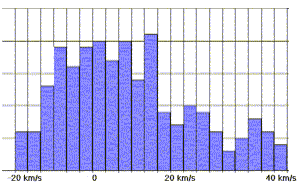Fig. 4. Ethereal pseudo-gas velocity distribution Besides, having built histogram of ethereal pseudo-gas velocity distribution, we can see that it close to Poisson distribution (refer to fig.4), usually appearing at dissipation of counter flows of particles. Ethereal pseudo-gas is leding away by gravity and other power in the ether. This is seen from velocity distribution. For instance, maximum velocity to the Earth equals the second space velocity of Solar system. Its contribution to gravitation phenomena is measly.

Statics

One of the main ether property is practically absolute elasticity . This property is similar to physical medium, which itself generates, - gaseous, liquid and solid. Being carrier of the whole energy of the Universe, ether already inheres in the state of isotropic compression that is expressed in presence its maximum fixed potential at absence of gravitating bodies: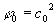[m2/s2], (2)

here c0 - is wave velocity within ether, far from gravitating mass.

At velocities to much smaller than light velocity and on distances much smaller than cosmological, potential of ether compression in presence of gravitating bodies is described by known scalar field in differential equation of Poisson: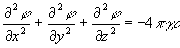[1/s2], (3)

here γ - is gravity constant [m3/(kg s2)],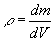- is volume density of mass distribution [kg/m3].

The Gradient of potential defines the gravity field tension (free fall acceleration) in given point of space: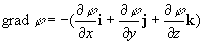[m/s2], (4)

here i, j, k - are orths of three-dimensional space.

Decision of equation (3) is: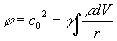[m2/s2], (5)

here r - is distance from measured points to gravitating volume dV.

Thence the law of universal gravitation is derivated by known way, diversified from classical by only cosmological adjustment, taking into account relaxation of ethereal vacancies: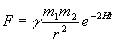[kg m/s2], (6)

here F - is gravity force,
γ - is gravity constant [m3/(kg s2)],
m1 , m2 - is gravitating masses [kg],
r - is distance between bodies [m],
H - is Hubble constant [1/s],
t - is a light distance between gravitating bodies [s].

The exponential multiplier in (6) excludes Zeeliger gravity paradox, providing exception influence of infinite remote masses.

Kinematics

The variable member of potential (5), equal square of the second space velocity, that is velocity required for remoting from gravitating masses to the infinity, for spherical gravitating mass is: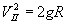[m2/s2],

here g - is a free fall acceleration,
R - is a radius of gravitating mass.

For instance, for the Earth VII = 11.2 [km/s]. Thence is possible to find the difference of velocities of waves (the light) within ether (on the Earth's orbit) in absence of gravitation and on surfaces of the Earth: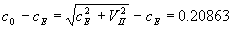[m/s], (7)

Obliquely changing waves velocities within ether in consequence of gravity potential fall is fixed in 1959 by Pound and Rebka used Mossbauer effect . Under 22.6-metre difference of heights of location of source and absorber of gamma-radiation, relative difference of frequencies was 2.5·10-15 .

In this experiment an gravity potential increment to account differences of heights was:

Δφ = gE Δh = 9.8 · 22.6 = 221.48 [m2/s2],

here gE - is the free fall acceleration on the Earth surface [m/s2].

Ditto increment on measurement differences of frequencies gives: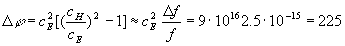[m2/s2]

It is seen that Pound-Rebka experiment well fits with ethereal theory of Universe. Besides that experiment in 1971 there was organized more exact experiment with using of missile with atomic clock on board, provided much greater difference of heights, given results which exactly correspond to the ethereal theory.

It is known that general mechanical energy of body consists of two forms: potential Wp and kinetic Wk.

W = Wp + Wk [kg m2/s2].

The ether is source of whole existing energy in Universe. Being Kept in them in the potential (1). The energy is realized under spontaneous birth the elementary particles, which are owners of potential energy Wp at birth.

During itheir drop in gravity field pits this energy is converted in kinetic to account of growing of velocity of this mass:

dWk = m VdV ; Wk = mV2/2 [kg m2/s2].

Naturally, the initial potential energy gets lost on this value.

Dynamics*

We will expect that, according to working model, particle, having a mass, emits gravitons with frequency, corresponding to (1), as follows, periodically excitating around itself phase ether with intensity, for instance, under the law:

 I = A0 cos2(ωt), ω = 2πmc2/h , [s-1] (8)

here ω - is the circular frequency of graviton emission.

Then, during a motion of this mass across the phase ether with the speed of v = dx/dt , the interaction between phase fronts excitated at present moment by wave I and excitated on dt before wave Id will be occur under the law:

dA = I + Id = A0 cos2[ωt-(k-dk)x] + A0 cos2[(ω - dω)t - (k+dk)x]=

= A0 {1+ cos[2ωt-2(k-dk)x] / 2 + cos[(2ωt-2)t-2(k-dk)x]} / 2 =

= A0 {1+cos(dωt - dkx) cos[(ω+dω)t]},              (9)

Since velocity of waves propagation is velocity of light, then from (8) and (9) follows that

dω = 2πmvc/h [s-1], dk =2πmv/h [m-1].

It is seen that got "beating" of ethereal vacuum, caused by gravitons waves, there is nothing else than de Broglie waves.

We will Expect that outside force F acts on body (the particle). Then, according to (8) counteracting outside power F asymmetry of gravity field density will appear around that particle, and it will equal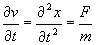[m/s2], (10)

It is seen that as gravity field gradient (4), so and gravity acceleration (10) have the same reason - asymmetry of gravity field. Difference consists only in following. Free fall acceleration appears from asymmetry of distant gravitational field ("macro fild"), but inertional acceleration appears from gradient around elementary particle ("micro field").

Value of Light Velocity out of Gravitation**

Using formula (7) it is possible to find "true" value of light velocity in Universe out of gravitation. For that lets consider the hierarchy of gravitational pits - potentials in observed Space (fig.5).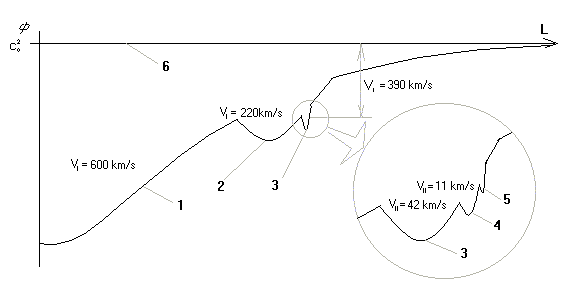Fig. 5. Structure of gravitational pits in Universe.
(1 - gravity pit of Great Attractor, 2 - gravity pit of Galaxy,
3 - gravity pit of Sun, 4 - Earth, 5 - Moon, 6 - level of zero gravity)

Knowing that velocity of Earth's movement within corpuscular ether is 390±30 km, (see, for example, Klimushkin D.J. Cosmology, 6.2. Pecular velocity of galaxies), that naturally to take it as first Space velocity for Solar system comparatively of Great Attractor, and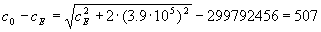[m/s], (11)

Value (11) much more (7) and it is necessary to take into account in fine analysis of spectrums of cosmologically distant objects. Thereby value of light velocity in absence of gravity is 299792963 [m/s].

For ether, either as for any elastic mediums, it is possible to calculate the fictituous Young's modulus by using volume (11).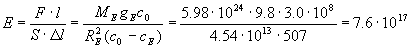[kg/(m s2)],

here ME - is the Earth mass [kg],
RE - is the Earth radius [m].

We will notice that real Young's modulus for ether without vacancies is equal infinity.

Conclusions

1. The ether is base of Universe. All the rest are only measly phenomenas inside itself.
2. It is possible to divide the ether on two types:
• the main, consisting of dense packed incondensable elements, being carrier of corpuscular (group) motion, and practically still in cosmological scale, original pseudo-solid;
• the ether of vacancies, consisting of empty cells of the ether of the main type, led away by mass bodies, original ethereal pseudo-gas, defining wave characteristics of motion (the phase velocity).

3. The gravitation phenomena is radiation by bodies, having stationary mass, vacancies of the ether - "gravitons" and, as effect of this, ether decompaction. It explicable without leaning on the nothing (empty, relative and abstract space).
4. The phenomena of reduction of the light velocity under gravity influence is explicable without breach of laws of conservation of energy, masses, constancies of time current or need of space and logic distortions.
5. The Universe is greatly deterministic and discrete. The traces from any most measly event: moving of electron, thoughts are scattering at the light velocity, but saving in the ethereal vacancies with stable paths during millions years.
6. The de Broglie waves are gravitational waves on the micro-scale level.
7. The gravitational force and inertial force have one general reason - asymmetry of gravitational field. The difference is only in a space scale.

References

1. Khaidarov K.A. Eternal Universe. - Borovoye, 2003.
2. Hooke R. An Attempt to Prove the Motion of the Earth by Observations, London, 1674
3. Ritz W. - Gesammelte Werke. Walter Ritz. Oeuvres - Paris: Gautier-Villars, 1911.
4. Gorbatsevich F.F. - Theory of non-empty ether. - Appatites, 1998.
5. Pound R.V., Rebka G.A. - Phys.Rev.Lett., 4 (1960) 337.

Karim Khaidarov
Borovoye, September 1, 2003.
Correction and additions - Sept 11, 2003.
* added on Sept 16, 2003
** added on Oct 16, 2003main page   list of papers   library